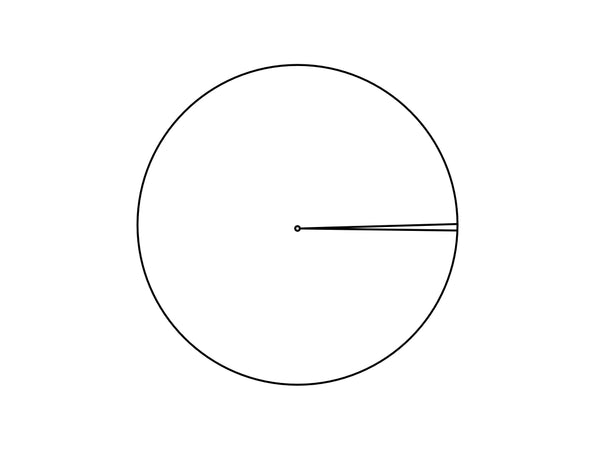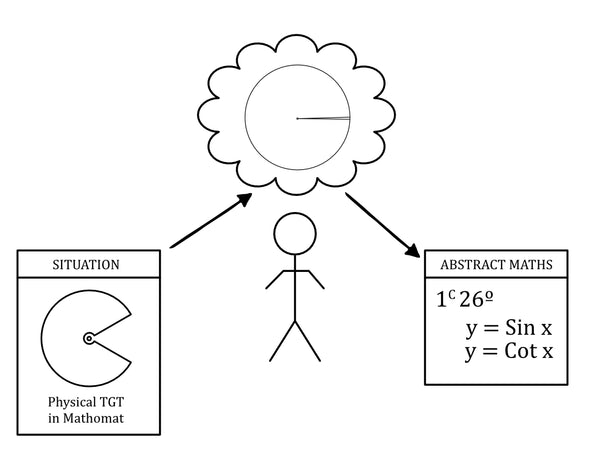See The New Mathomat R300 Ruler

# A theoretical model for the use of Mathomat in the Platonic protractor activities

The angles section of the Explorer manual asks students to develop a mental image of angle that is illustrated in figure 1. This involves two arms, a vertex, and an opening. Mike Mitchelmore and Paul White (2018) refer to this geometric object as the standard angle concept.

Figure 1The standard angle concept
Source: Mitchelmore and White (1998)

In the introduction to his PhD thesis (2018) Hamilton Hardison presented two models for reasoning about a one-degree angle. These are shown below in figures two and three. Both models produce valid results, however they also both represent entirely different ways for reasoning about angle. In figure two the standard angle concept is centered in an arbitrary circle, and it intercepts the circumference with a length equal to 1/360th of the circle’s circumference. In figure three the one-degree angle radially partitions the plane into 360 equiangular parts and selects one of them.

Figure 2Circular model for a one-degree angle
Source: Hardison (2018, p.3)

Figure 3A model for a one-degree angle produced by partitioning the plane
Source: Hardison (2018, p,3)

Angle as a quantity is argued in Hardison’s (2018) thesis to be a mental construct. It needs to be emphasised that every person creates their mental construct in their own unique way. None the less Hardison’s research builds on the work of researchers such as Moore (2013), mentioned earlier, to argue that in secondary school students need to develop an ability to reason about angle using the circular model shown in figure two. Being able to measure angle as a ratio of arc lengths reflects a robust understanding of it as a concept and supports success with the more abstract mathematical concepts encountered by secondary students such as trigonometry and calculus.

The TGT in Mathomat has the same structure as the model in figure two. In my opinion it is a reification of this model, being it’s physical representation. This is neither surprising nor coincidental. As he designed the TGT, Chris Tisdell was wrestling with the task of capturing the ability of a compass and straight edge to explore geometric structure, he reduced these tools to their primitive elements of vertex, line and arc and then reassembled them in a more actionable, integrated, form.

The similarity between the TGT, and the circular model for angle in figure two, means that we can assemble them within the modelling framework described below in figure four by John Mason and J Davis (2011).  This results in the theoretical model for describing how the TGT in Mathomat works during the Plato’s protractor activities described in figure five.

Figure 4Illustration of the modelling process
Source: Mason and Davis (1991, p.11)

Figure 5The Mason and Davis modelling process adapted for circular models of angle and the TGT
Source: John Lawton 2022

The circular, mental, model of angle in figure five is manipulated by students to make sense of their physical world. As Mason and Davis (2011 , p9) point out, the transition from mental world to mathematical world involves a process called mathematisation. By positioning the TGT as a physical representation of the circular model for angle in student’s physical world we can connect their full range of senses to the task of operating the standard angle concept, but this time as a circular model involving a ratio of arc lengths. This works very effectively in Plato’s protractor 1, where students are asked to use their TGT to see an angle’s opening as openness, and in Plato’s protractor III where students are asked to operate mentally on their TGT by changing and measuring its amount of openness.

The concept of mathematisation was emphasised by Freudenthal (1968, 1991) as a way of explaining how students need to move from understanding real problems to developing a mathematical understanding for them. Mathematisation is central to the foundational work on angle by Mitchelmore and White (1998, 2000) who describe how the standard angle concept emerges from student’s physical experience. Plato’s protractor investigations use the model described in figure five to explain how students can transition from using a concept of angle as a stand-alone geometric object (the standard angle concept) to one based on a circular model that relates angle to arc length, through mathematisation.